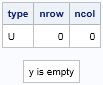An empty matrix is a matrix that has zero rows and zero columns. At first "empty matrix" sounds like an oxymoron, but when programming in a matrix language such as SAS/IML, empty matrices arise surprisingly often.

Sometimes empty matrices occur because of a typographical error in your program. If you try to print or compute with a matrix that has not been defined, the SAS/IML program will usually display an error message:

```proc iml; print UndefinedMatrix;   ERROR: (execution) Matrix has not been set to a value.```

However, sometimes an empty matrix is the correct result of a valid computation. For example:

• If you use the LOC function to find elements in a vector that satisfy a criterion, the empty matrix results when no elements satisfy the criterion:
```x = {2 4 6}; y = loc(x < 0); /* y is empty matrix */```
• If you use the XSECT function to find the intersection of two sets, the empty matrix results when the intersection is empty:
`y = xsect({1}, {2}); /* empty intersection of sets */`
• If you use the REMOVE function to remove elements of a matrix, the empty matrix results when you remove all elements:
`y = remove({2}, 1); /* remove 1st element from 1x1 matrix */`

In my book Statistical Programming with SAS/IML Software, I mention that you can determine whether a matrix is empty by using one of three functions: TYPE, NROW, or NCOL, as follows:

```print (type(y))[L="type"] (nrow(y))[L="nrow"] (ncol(y))[L="ncol"];   if ncol(y)=0 then print "y is empty"; else print "y is not empty";```The output shows that the "type" of an empty matrix is 'U' (for "undefined"). In my book I use the NCOL function to test for empty matrices, but the other functions work just as well.

### New function for detecting empty matrices

Recent releases of SAS/IML software support new ways to create and detect empty matrices:

• In SAS/IML 12.1, you can use curly braces to define an empty matrix:
`y = {}; /* y is an empty matrix */`
• In SAS/IML 12.3, you can use the ISEMPTY function to test whether a matrix is empty:
```if IsEmpty(y) then print "y is empty"; else print "y is not empty";```

I think that the new syntax produces SAS/IML programs that are easier to read and understand. Have you encountered empty matrices in your SAS/IML programs? Where? How did you handle them? Leave a comment.

ShareDistinguished Researcher in Computational Statistics

Rick Wicklin, PhD, is a distinguished researcher in computational statistics at SAS and is a principal developer of SAS/IML software. His areas of expertise include computational statistics, simulation, statistical graphics, and modern methods in statistical data analysis. Rick is author of the books Statistical Programming with SAS/IML Software and Simulating Data with SAS.

1.